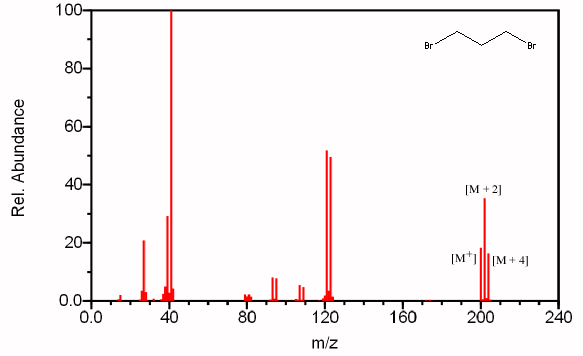What identifying characteristics would be present in the mass spectrum of a compound containing two bromine atoms?

Aug 15, 2016

There should be M, M+2, and M+4 peaks with relative intensities of 1:2:1.

Explanation:

For example, see the mass spectrum of 1,3-dibromopropane.Natural bromine is a mixture of 50.50 % $\text{^79"Br}$ and 49.50 % $\text{^81"Br}$.

Thus, the molecule could contain two $\text{Br-79}$ atoms, one $\text{Br-79}$ and one $\text{Br-81}$ atom, or two $\text{Br-81}$ atoms.

This will give rise to three molecular ion peaks, each separated by two mass units.

We can calculate the relative size of each peak.

M peak:

Probability of $2 \text{Br-79" = 0.5050^2 "(one way)} = 0.2550$

M+2 peak:

Probability of $\text{1 Br-79 and 1 Br-81" = 2(0.5050)(0.4950) "(two ways)} = 0.5000$

M+4 peak:

Probability of $\text{2 Br-81" = 0.4950^2 "(one way)} = 0.2450$

If we set the relative probability of the largest peak at 100, the relative intensities become

"M:M+2:M+4" = 51:100:49 ≈ 1:2:1

Other feature:

You should see a peak at M-79 (loss of $\text{Br}$).

Compare the peak at 121 in the spectrum of 1,3-dibromopropane,